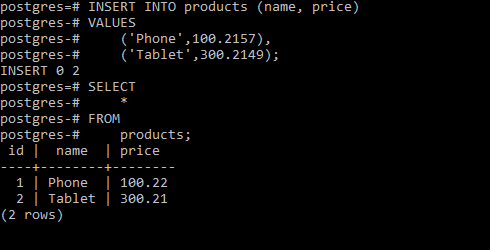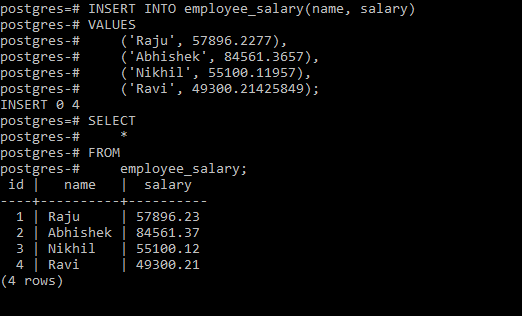# PostgreSQL – NUMERIC Data Type

• Last Updated : 28 Aug, 2020

PostgreSQL supports the `NUMERIC `type for storing numbers with a very large number of digits. Generally `NUMERIC` type are used for the monetary or amounts storage where precision is required.

```Syntax: NUMERIC(precision, scale)

Where,
Precision: Total number of digits.
Scale: Number of digits in terms of a fraction.```

The` NUMERIC` value can have up to 131, 072 digits before the decimal point of 16, 383 digits after the decimal point.
It is allowed to have a zero or positive scale, as the syntax defined below for a NUMERIC column with the scale of zero:

`Syntax: NUMERIC(precision)`

If you eliminate both precision and scale, there is no limit to the precision or the scale and the syntax will be as below:

`Syntax: NUMERIC`

The NUMERIC and DECIMAL types are equivalent in PostgreSQL and upto the SQL standard.
It is recommended to not use the NUMERIC type, if precision is not required as the calculation on NUMERIC values is slower than integers, floats, and double precision.

Example 1:
Create a new table named products with the below commands:

```CREATE TABLE IF NOT EXISTS products (
id serial PRIMARY KEY,
name VARCHAR NOT NULL,
price NUMERIC (5, 2)
);```

Now insert some products with the prices whose scales exceed the scale declared in the price column:

```INSERT INTO products (name, price)
VALUES
('Phone', 100.2157),
('Tablet', 300.2149);```

As the scale of the price column is 2, PostgreSQL rounds the value 100.2157 up to 100.22 and rounds the value 300.2149 down to 300.21
The below query returns all rows of the products table:

```SELECT
*
FROM
products;```

Output:Example 2:
Create a new table named products with the below commands:

```CREATE TABLE IF NOT EXISTS employee_salary(
id serial PRIMARY KEY,
name VARCHAR NOT NULL,
salary NUMERIC (10, 2)
);```

Now insert some products with the prices whose scales exceed the scale declared in the price column:

```INSERT INTO employee_salary(name, salary)
VALUES
('Raju', 57896.2277),
('Abhishek', 84561.3657),
('Nikhil', 55100.11957),
('Ravi', 49300.21425849);```

As the scale of the price column is 2, PostgreSQL rounds the value 57896.2277 up to 57896.22 for Raju, the value 84561.3657 down to 84561.36 for Abhishek, the value 55100.11957 to 55100.12 for Nikhil and the value 49300.21425849 to 49300.21 for Ravi.
The below query returns all rows of the products table:

```SELECT
*
FROM
employee_salary;```

Output:My Personal Notes arrow_drop_up